# Algebra II - Properties of Functions Review: Tutoring Solution Chapter Exam

Exam Instructions:

Choose your answers to the questions and click 'Next' to see the next set of questions. You can skip questions if you would like and come back to them later with the yellow "Go To First Skipped Question" button. When you have completed the practice exam, a green submit button will appear. Click it to see your results. Good luck!

### Page 1

#### Question 1 1. What is the range of the function represented by the graph below?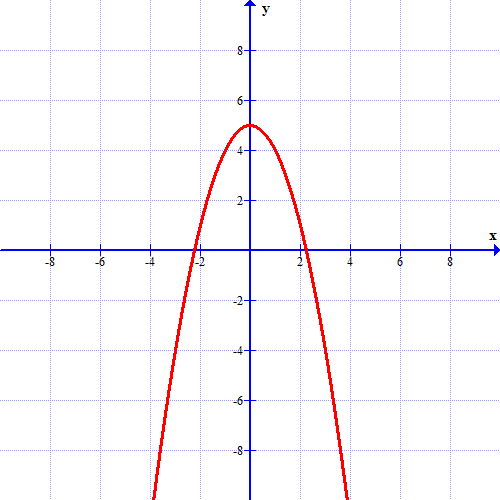#### Question 2 2. Using the graph, find f(f(-2)).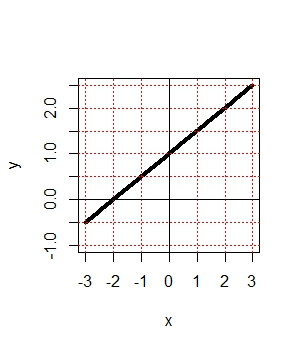### Page 2

#### Question 6 6. If the graph of f(x) is shown below, which choice represents the graph of f(x + 1) + 2?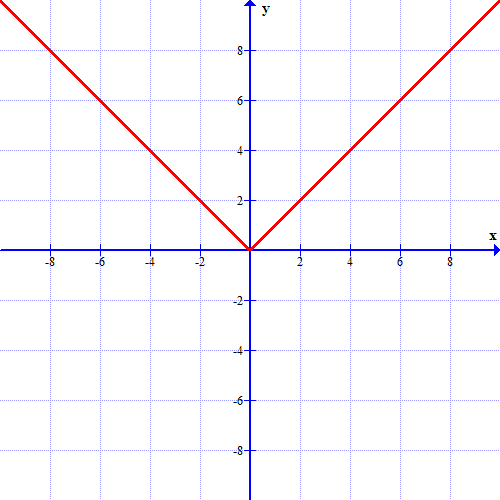#### Question 7 7. What is the domain of the function below?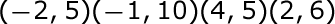### Page 3

#### Question 12 12. Use the graph to find f(f(2)).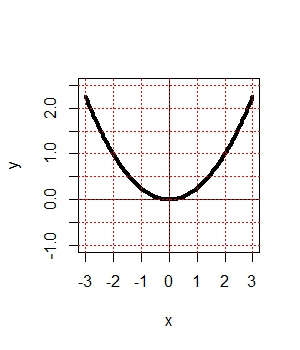### Page 5

#### Question 22 22. In the figure shown below, the graph of the yellow function is a translation of the graph of the blue function. If the expression for the blue function is y(x) = b(x), which of the following is the equation of the yellow function?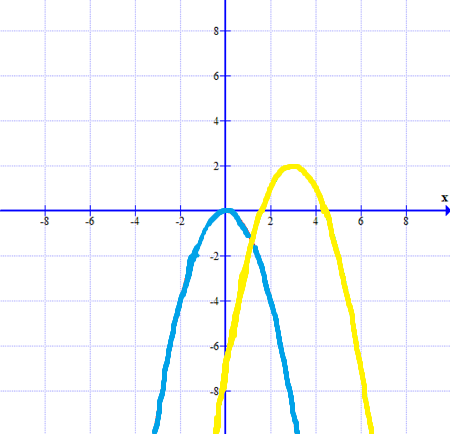### Page 6

#### Question 26 26. Which kind of transformation would change the purple function below into the yellow one?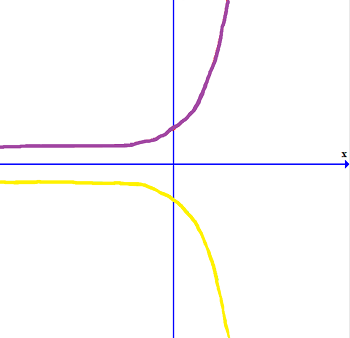#### Question 30 30. What is the inverse function of f(x) = 3(x - 2)3?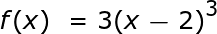#### Algebra II - Properties of Functions Review: Tutoring Solution Chapter Exam Instructions

Choose your answers to the questions and click 'Next' to see the next set of questions. You can skip questions if you would like and come back to them later with the yellow "Go To First Skipped Question" button. When you have completed the practice exam, a green submit button will appear. Click it to see your results. Good luck!

Support